### ROUND Function Examples – Excel, VBA, & Google Sheets

This tutorial demonstrates how to use the Excel ROUND Function in Excel to round a number.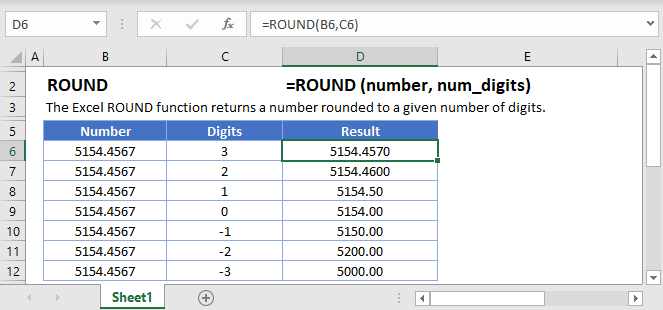## ROUND Function Overview

The ROUND Function Rounds a number to a specified number of digits.

To use the ROUND Excel Worksheet Function, select a cell and type: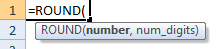(Notice how the formula inputs appear)

### ROUND Function Syntax and Inputs:

`=ROUND(number,num_digits)`

number – A number.

num_digits – The number of decimal places to round. Negative rounds to the left of the decimal.

AutoMacro - VBA Code Generator

## How to use the ROUND Function

### Round to Nearest Whole Number

To round to the nearest whole number set the num_digits argument to 0.

`=ROUND(A2,0)`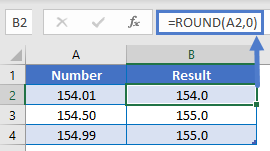### Round to Two Decimal Places

To round to two decimal places, set the num_digits argument to 2. Here we will round a price to the nearest cent:

`=ROUND(B3,2)`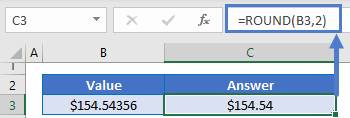### Round to Nearest 10, 100, or 1000

To round to the other side of the decimal (to 10, 100, or 1000s), use negative numbers:

`=ROUND(A2,-2)`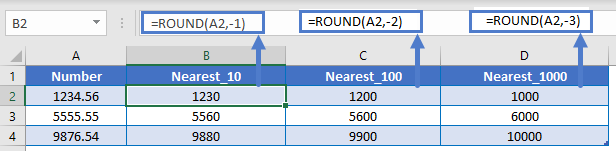### Round Percentages

When rounding percentages remember that percentages are decimal values. So to round to the nearest whole percentage use the ROUND Function with num_digits = 2.

`=ROUND(A2,2)`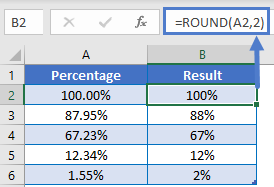### Round to n Significant Figures

If you need to round a number to n number of significant digits use this formula (click the link to learn more about why this formula works):

`=ROUND(A2,B2-(1+INT(LOG10(ABS(A2)))))`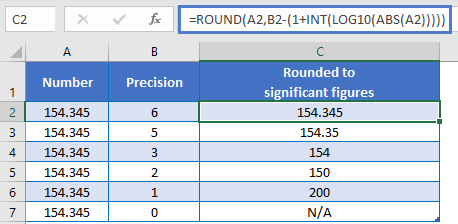## Other ROUND Functions / Formulas

Excel / Google Sheets contains many other round functions. Here are quick examples of each: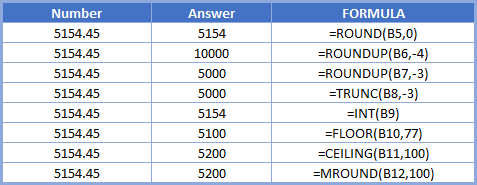Read our tutorials to learn more:

Below, we will point out a few functions in particular.

### ROUNDUP and ROUNDDOWN

The ROUNDUP and ROUNDDOWN Functions work exactly the same way as the ROUND Function, except they always round up or down.

```=ROUNDUP(A2,-2)
=ROUNDDOWN(A2,-2)```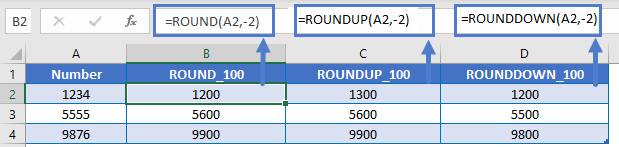### ROUND to Multiplies

ROUND, ROUNDUP, and ROUNDDOWN round to certain number of decimal places. Instead you can round to multiples by using the MROUND, FLOOR, CEILING Functions.

```=MROUND(B3,5)
=FLOOR(B3,5)
=CEILING(B3,5)```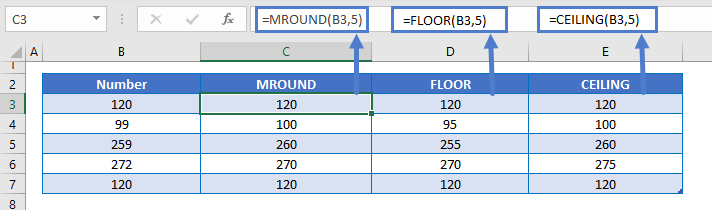## Round in Google Sheets

All of the above examples work exactly the same in Google Sheets as in Excel.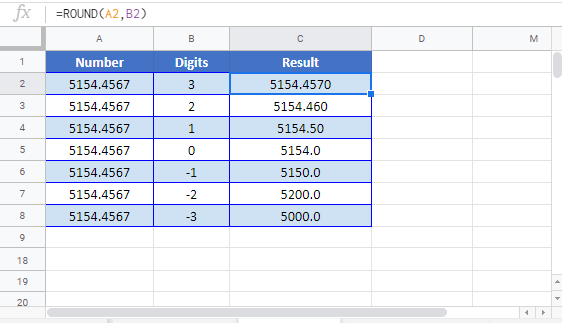## ROUND Examples in VBA

You can also use the ROUND function in VBA. Type:
`application.worksheetfunction.round(number,num_digits)`
For the function arguments (number, etc.), you can either enter them directly into the function, or define variables to use instead.

## Excel Practice Worksheet

Practice Excel functions and formulas with our 100% free practice worksheets!

• Automatically Graded Exercises
• Learn Excel, Inside Excel!